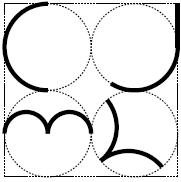[contest dates] Contest 1: 10/20/2018 [calc-ok] Contest 2: 12/08/2018 [no-calc] Contest 3: 5/11/2019 [calc-ok] [location] The meet will be held at two sites, Whitney Young and Lane Tech, with the exception of the final meet, which will be held at Lane Tech. [contest topics] CONTEST 1 - calc-ok SCHEDULE * Individual Round: 20 questions in 50 minutes, one point per question * Team Round (Candy Bar): 20 questions in 50 minutes TOPICS * 6th grade: ratio, proportion and percent, geometry: area and perimeter of circles, rectangles and triangles * 7th grade: basic statistics (arithmetic mean, median, mode); divisibility (primes, composites, GCD, LCM, relatively prime, number of divisors, etc.); ratios, proportions, and percents * 8th grade: basic statistics (arithmetic mean, median, mode, stem and leaf plots, box and whiskers plots); divisibility (primes, composites, GCD, LCM, relatively prime, number of divisors, etc.); arithmetic and geometric sequences (including partial sums) CONTEST 2 - no-calc SCHEDULE * Individual Round: 20 questions in 50 minutes, one point per question * Team Round (Candy Bar): 20 questions in 50 minutes TOPICS * 6th grade: basic geometry (angle measurement, perimeter, area, volume), probability (and/or easy inclusion/exclusion), counting (permutations and combinations), the Pythagorean Theorem * 7th grade: basic geometry (angle measurement, perimeter, area, volume), probability (and/or easy inclusion/exclusion), counting (permutations and combinations), the Pythagorean Theorem * 8th grade: basic geometry (angle measurement, perimeter, area, volume, polygon formulas), probability (and/or easy inclusion/exclusion), counting (permutations and combinations), the Pythagorean Theorem (including special triangles, 30-60-90 and 45-45-90) CONTEST 3 - calc-ok SCHEDULE * Individual Round: 20 questions in 50 minutes, one point per question * Team Round (Candy Bar): 20 questions in 50 minutes TOPICS * 6th grade: linear equations, number patterns, geometric similarity, interpreting graphs * 7th grade: linear equations, number patterns, geometric similarity, interpreting graphs * 8th grade: systems of linear equations, number patterns, symmetry and transformations, quadratic equations [contest formats] I. Individual Contests — Younger students may compete at a higher grade level. Each grade level contest may have as many as six contestants and any number of alternates.II. Contests 1 and 3 are calc-okay, Contest 2 is not.
admin · about cjml ·faq · contact us